Latest Banking jobs   »   Quantitative Aptitude Quiz For IBPS RRB...

# Quantitative Aptitude Quiz For IBPS RRB PO, Clerk Prelims 2021- 30th May

Directions (1-5): What approximation value should come in the place of question mark.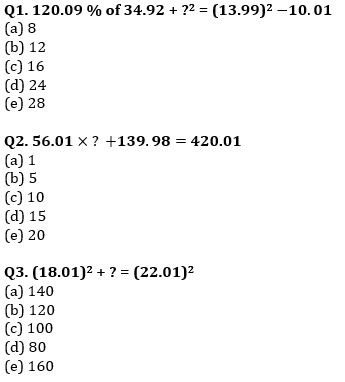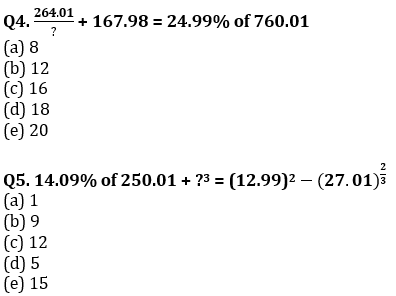Directions (6-11): Given below pie chart show percentage distribution of accounts opened by five different banks under ‘Jan Dhan Yojana’. Read the data carefully and answer the question.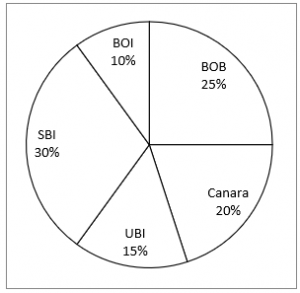Q6. If difference between total accounts opened by SBI and Canara is 2.1 cr, then find total account opened by UBI ?
(a) 2.15 cr
(b) 3.15 cr
(c) 3.25 cr
(d) 3.05 cr
(e) 3.75 cr

Q7. Find ratio between total accounts opened by SBI & BOI together to total accounts opened by Canara ?
(a) 2 : 1
(b) 3 : 1
(c) 4 : 1
(d) 5 : 1
(e) 1 : 1

Q8. Total accounts opened by BOB is what percent more than total accounts opened by BOI ?
(a) 200%
(b) 125%
(c) 75%
(d) 100%
(e) 150%

Q9. If total accounts opened by UBI and BOI is 3.6 cr, then find difference between accounts opened by Canara and BOB ?
(a) 0.64 cr
(b) 0.72 cr
(c) 0.56 cr
(d) 0.48 cr
(e) 0.42 cr

Q10. If total 24 cr accounts opened by all five bank then find average number of accounts opened by SBI and UBI ?
(a) 5.6 cr
(b) 5.4 cr
(c) 5.2 cr
(d) 6.4 cr
(e) 7.2 cr

Q11. Find total accounts opened by BOB is what percent less than total accounts opened by SBI ?
(a) 20%
(b) 10%
(c) 12 ½%
(d) 14 2/7%
(e) 16 ⅔%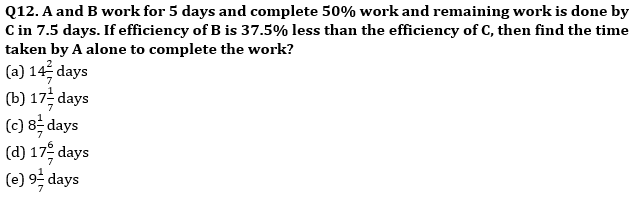Q13. An amount is invested at Simple Interest for 2 years and after two years whole amount (Initial amount+Intrest ) is invested at Compound Interest for 2 more years. If rate of interest is 10% for both simple and compound interest and total interest earned after 4 years is 1130Rs. then, find the initial amount invested?
(a) 2500
(b) 3000
(c) 1500
(d) 1880
(e) 2340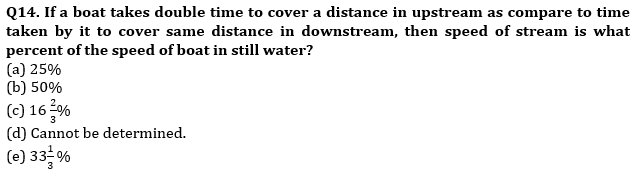Q15. Two jar A and B contain a mixture of two liquids, X and Y in the ratio of 2 : 3 and 4 : 5 respectively. If mixture from jar A and B are mixed in the ratio of 1 : 2 then, find the ratio of X and Y in final mixture.
(a) 70:51
(b) 58:77
(c) 61:77
(d) 77:58
(e) 62:61

Directions (16-20): In the following two equations questions numbered (I) and (II) are given. You have to solve both equations and Give answer
(a) If x > y
(b) If x ≥ y
(c) If y > x
(d) If y ≥ x
(e) If x = y or no relation can be established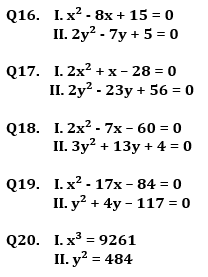Practice More Questions of Quantitative Aptitude for Competitive Exams:

###### Study Plan for IBPS RRB PO/Clerk Prelims 2021

Solutions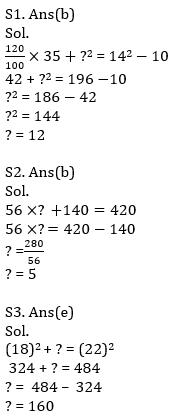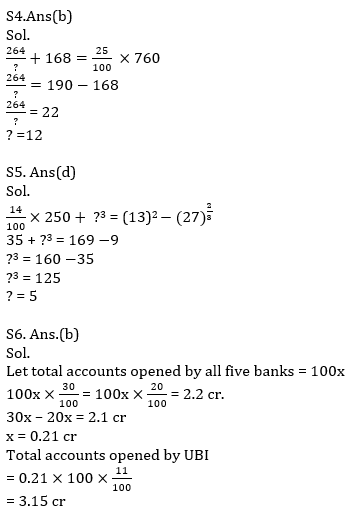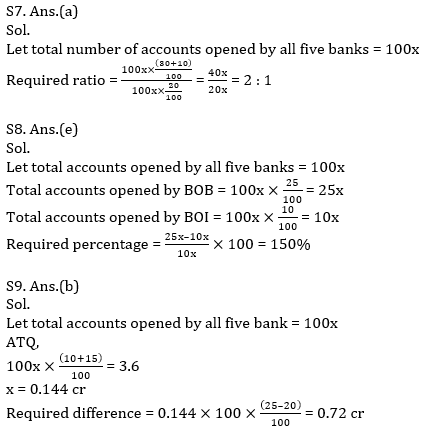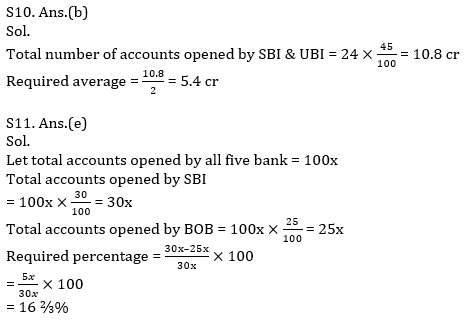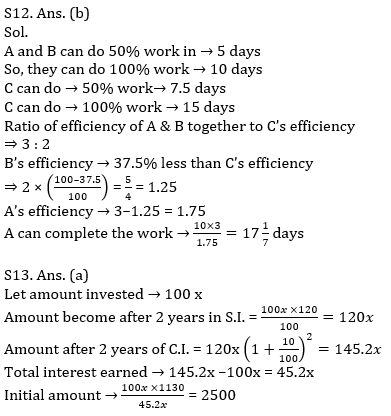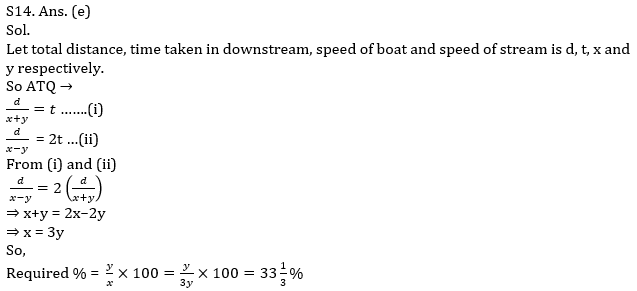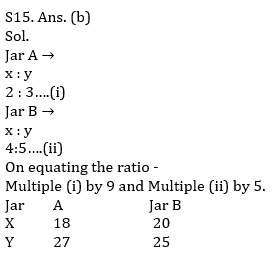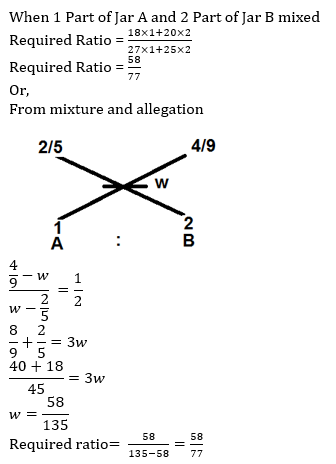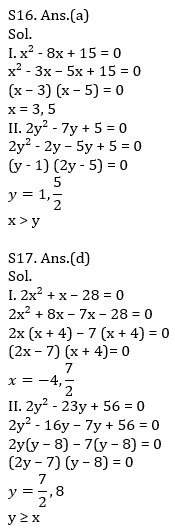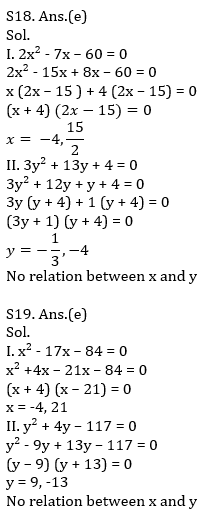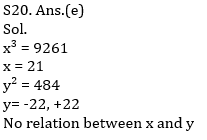#### Congratulations!Incorrect details? Fill the form again here

•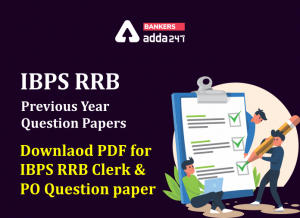IBPS RRB Previous Year Question Paper PD...
•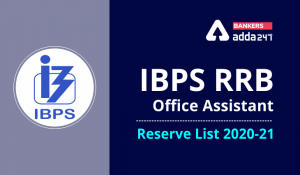IBPS RRB Clerk 2nd Reserve List 2022 Out...
•Quantitative Aptitude Quiz For IBPS RRB ...
•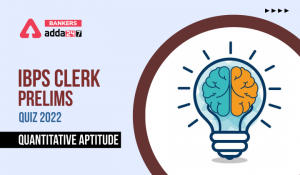Quantitative Aptitude Quiz For IBPS Cler...
•Reasoning Ability Quiz For IBPS RRB PO P...
•Quantitative Aptitude Quiz For IBPS RRB ...